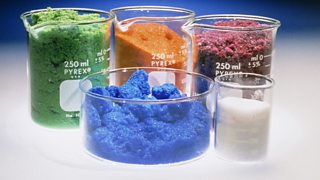# The mole

Chemists measure the amount of a substance in a unit called ‘the mole’. This is a convenient way of counting . It allows chemists to make predictions about the of different substances that are involved in reactions.

One mole is the Avogadro number of particles (atoms, , or ) in a substance.

One mole of atoms contains 6 x 1023 atoms, no matter what it is. This is a very large number: it is 6 with 23 zeros after it. It is known as the Avogadro number.

This number is used in chemistry because if you could count out this many carbon atoms, the total mass of carbon you would have is 12 g. On the other hand, weighing out 12 g of carbon allows you to know how many atoms you have.

## Moles of elements

One mole of carbon atoms has a mass of exactly 12 g. Because magnesium atoms each have twice the mass of carbon atoms (24Mg compared with 12C), one mole of magnesium has a mass of 24 g. In fact, one mole of any element has a mass in grams that is equal to its relative atomic mass. One mole of iron has a mass of 56 g.

## Moles of compounds

A mole of a molecular compound contains 6 x 1023 molecules. It has a mass that is equal to its . So a mole of water (H2O) has a mass of 18 g. A mole of carbon dioxide (CO2) has a mass of 44 g. This also works for ionic compounds, so a mole of sodium chloride (NaCl) has a mass of 58.5 g.

This approach can also be used for elements that are made from molecules. For example, oxygen gas O2 is diatomic (each molecule contains two atoms) so its relative formula mass is 32. One mole of oxygen molecules would therefore have a mass of 32 g. One mole of oxygen atoms (if you could ever isolate them) would have a mass of 16 g.Varying volume of substances